# 445615212-SOLVES-ROUTINE-NON-ROUTINE-PROBLEM-INVOLVING-THE-VOLUME-OF-THE-RECTANGULAR-PRISM-pptx.pptx

1. Jun 2023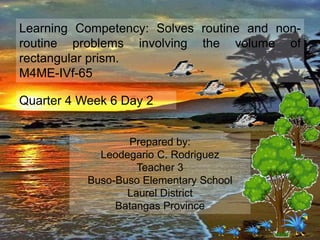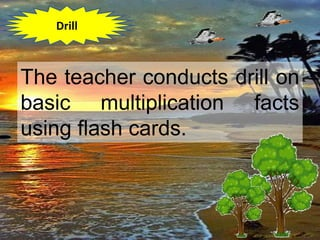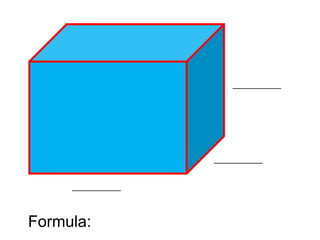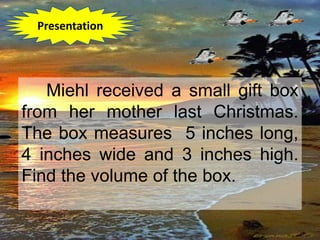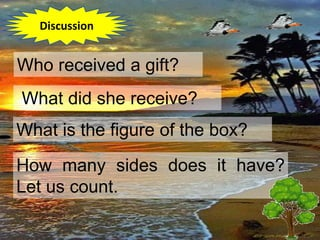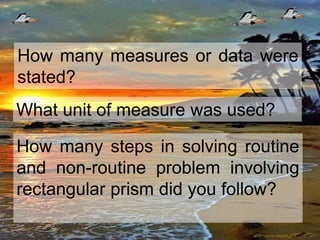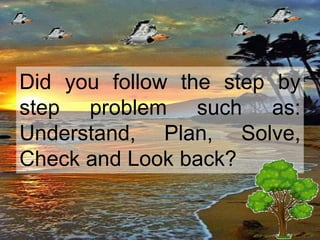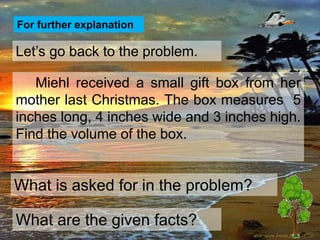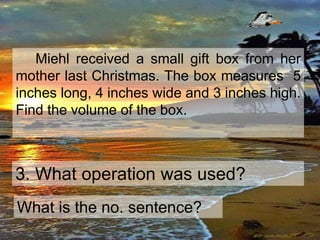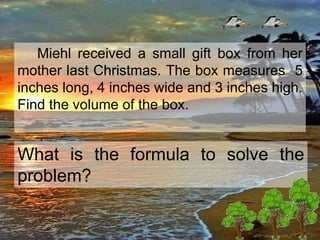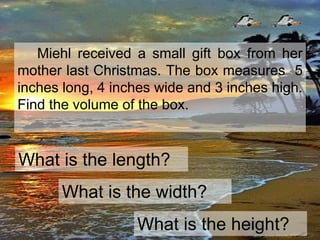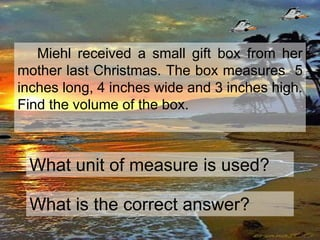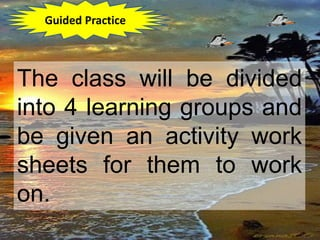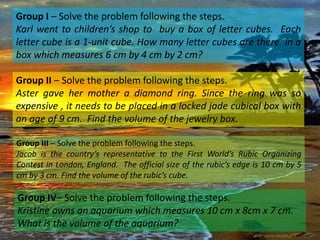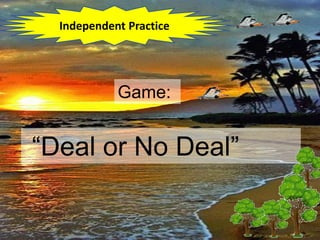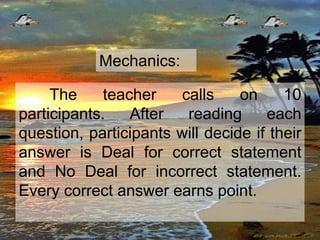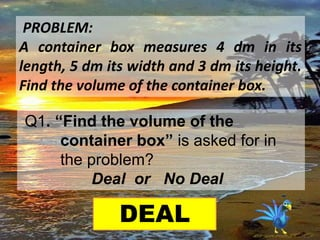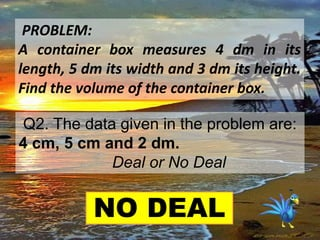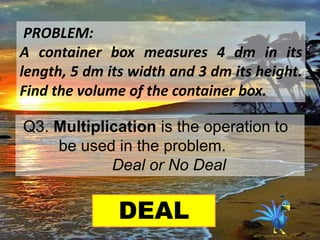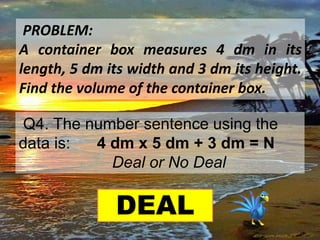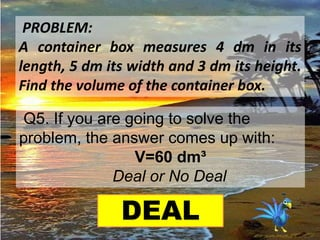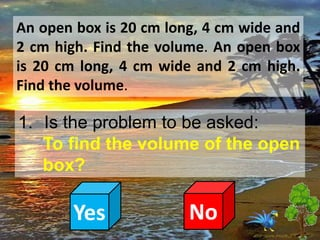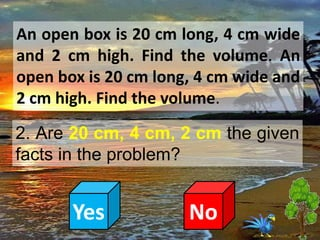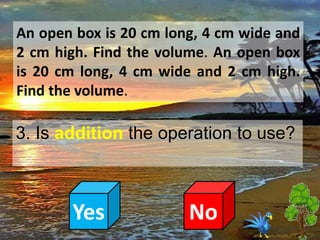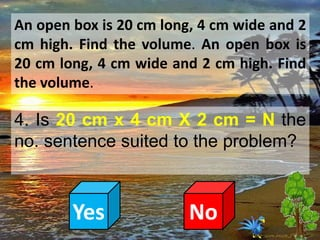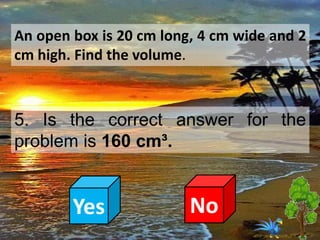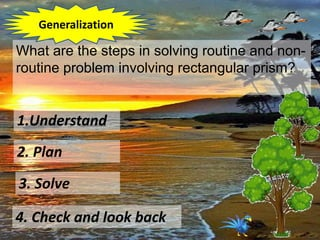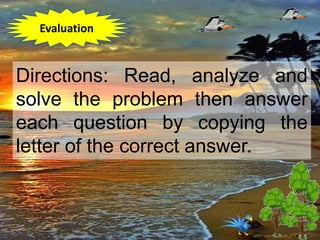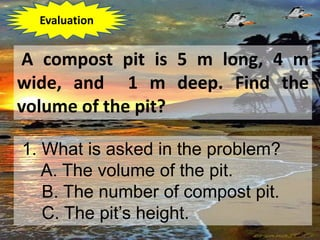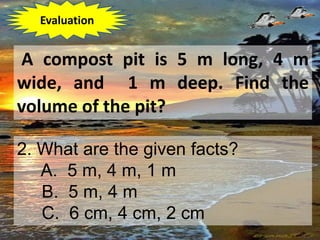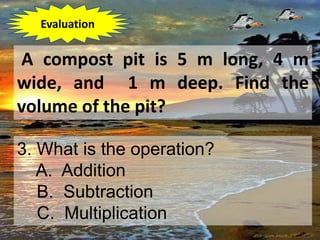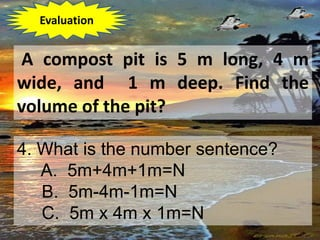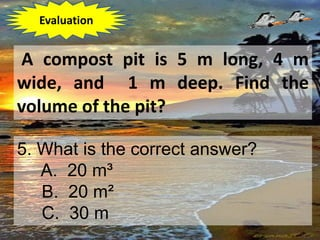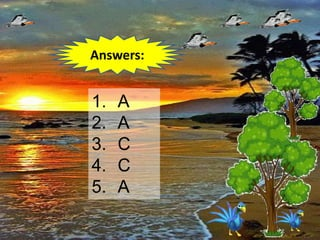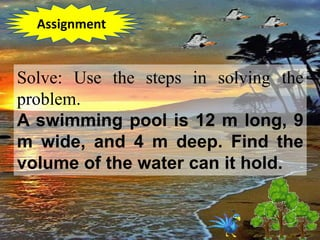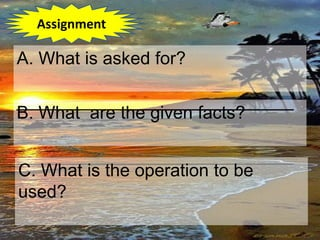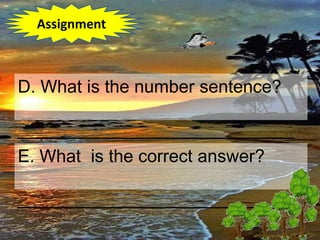1 von 57

### 445615212-SOLVES-ROUTINE-NON-ROUTINE-PROBLEM-INVOLVING-THE-VOLUME-OF-THE-RECTANGULAR-PRISM-pptx.pptx

• 1. Learning Competency: Solves routine and non- routine problems involving the volume of rectangular prism. M4ME-IVf-65 Quarter 4 Week 6 Day 2 Prepared by: Leodegario C. Rodriguez Teacher 3 Buso-Buso Elementary School Laurel District Batangas Province
• 2. The teacher conducts drill on basic multiplication facts using flash cards. Drill
• 3. The teacher conducts review about the parts of the rectangular prism and its formula. Review
• 4. ___________ ___________ ___________ Formula:
• 5. Show a gift box having a rectangular figure. Motivation
• 6. Have you received a gift last Christmas? What was it? How many gifts did you receive? Who gave them to you? Motivation
• 7. *What did you say to the person who gave something for you? Is it good to be thankful to anybody? Why? Valuing
• 8. Miehl received a small gift box from her mother last Christmas. The box measures 5 inches long, 4 inches wide and 3 inches high. Find the volume of the box. Presentation
• 9. Let the pupils show the solution using the steps in solving problem.
• 10. Who received a gift? What did she receive? What is the figure of the box? How many sides does it have? Let us count. Discussion
• 11. How many measures or data were stated? What unit of measure was used? How many steps in solving routine and non-routine problem involving rectangular prism did you follow?
• 12. Did you follow the step by step problem such as: Understand, Plan, Solve, Check and Look back?
• 13. Let’s go back to the problem. For further explanation Miehl received a small gift box from her mother last Christmas. The box measures 5 inches long, 4 inches wide and 3 inches high. Find the volume of the box. What is asked for in the problem? What are the given facts?
• 14. Miehl received a small gift box from her mother last Christmas. The box measures 5 inches long, 4 inches wide and 3 inches high. Find the volume of the box. 3. What operation was used? What is the no. sentence?
• 15. Miehl received a small gift box from her mother last Christmas. The box measures 5 inches long, 4 inches wide and 3 inches high. Find the volume of the box. What is the formula to solve the problem?
• 16. Miehl received a small gift box from her mother last Christmas. The box measures 5 inches long, 4 inches wide and 3 inches high. Find the volume of the box. What is the length? What is the width? What is the height?
• 17. Miehl received a small gift box from her mother last Christmas. The box measures 5 inches long, 4 inches wide and 3 inches high. Find the volume of the box. What unit of measure is used? What is the correct answer?
• 18. The teacher will visualize the problem on the board.
• 19. The class will be divided into 4 learning groups and be given an activity work sheets for them to work on. Guided Practice
• 20. Group I – Solve the problem following the steps. Karl went to children’s shop to buy a box of letter cubes. Each letter cube is a 1-unit cube. How many letter cubes are there in a box which measures 6 cm by 4 cm by 2 cm? Group II – Solve the problem following the steps. Aster gave her mother a diamond ring. Since the ring was so expensive , it needs to be placed in a locked jade cubical box with an age of 9 cm. Find the volume of the jewelry box. Group III – Solve the problem following the steps. Jacob is the country’s representative to the First World’s Rubic Organizing Contest in London, England. The official size of the rubic’s edge is 10 cm by 5 cm by 3 cm. Find the volume of the rubic’s cube. Group IV– Solve the problem following the steps. Kristine owns an aquarium which measures 10 cm x 8cm x 7 cm. What is the volume of the aquarium?
• 21. Game: “Deal or No Deal” Independent Practice
• 22. The teacher calls on 10 participants. After reading each question, participants will decide if their answer is Deal for correct statement and No Deal for incorrect statement. Every correct answer earns point. Mechanics:
• 23. PROBLEM: A container box measures 4 dm in its length, 5 dm its width and 3 dm its height. Find the volume of the container box. Q1. “Find the volume of the container box” is asked for in the problem? Deal or No Deal DEAL
• 24. PROBLEM: A container box measures 4 dm in its length, 5 dm its width and 3 dm its height. Find the volume of the container box. Q2. The data given in the problem are: 4 cm, 5 cm and 2 dm. Deal or No Deal NO DEAL
• 25. PROBLEM: A container box measures 4 dm in its length, 5 dm its width and 3 dm its height. Find the volume of the container box. Q3. Multiplication is the operation to be used in the problem. Deal or No Deal DEAL
• 26. PROBLEM: A container box measures 4 dm in its length, 5 dm its width and 3 dm its height. Find the volume of the container box. Q4. The number sentence using the data is: 4 dm x 5 dm + 3 dm = N Deal or No Deal DEAL
• 27. PROBLEM: A container box measures 4 dm in its length, 5 dm its width and 3 dm its height. Find the volume of the container box. Q5. If you are going to solve the problem, the answer comes up with: V=60 dm³ Deal or No Deal DEAL
• 29. An open box is 20 cm long, 4 cm wide and 2 cm high. Find the volume. An open box is 20 cm long, 4 cm wide and 2 cm high. Find the volume. 1. Is the problem to be asked: To find the volume of the open box? Yes No
• 30. An open box is 20 cm long, 4 cm wide and 2 cm high. Find the volume. An open box is 20 cm long, 4 cm wide and 2 cm high. Find the volume. 2. Are 20 cm, 4 cm, 2 cm the given facts in the problem? Yes No
• 31. An open box is 20 cm long, 4 cm wide and 2 cm high. Find the volume. An open box is 20 cm long, 4 cm wide and 2 cm high. Find the volume. 3. Is addition the operation to use? Yes No
• 32. An open box is 20 cm long, 4 cm wide and 2 cm high. Find the volume. An open box is 20 cm long, 4 cm wide and 2 cm high. Find the volume. 4. Is 20 cm x 4 cm X 2 cm = N the no. sentence suited to the problem? Yes No
• 33. An open box is 20 cm long, 4 cm wide and 2 cm high. Find the volume. 5. Is the correct answer for the problem is 160 cm³. Yes No
• 34. What are the steps in solving routine and non- routine problem involving rectangular prism? 1.Understand 2. Plan 3. Solve 4. Check and look back Generalization
• 35. Directions: Read, analyze and solve the problem then answer each question by copying the letter of the correct answer. Evaluation
• 36. A compost pit is 5 m long, 4 m wide, and 1 m deep. Find the volume of the pit? 1. What is asked in the problem? A. The volume of the pit. B. The number of compost pit. C. The pit’s height. Evaluation
• 37. A compost pit is 5 m long, 4 m wide, and 1 m deep. Find the volume of the pit? 2. What are the given facts? A. 5 m, 4 m, 1 m B. 5 m, 4 m C. 6 cm, 4 cm, 2 cm Evaluation
• 38. A compost pit is 5 m long, 4 m wide, and 1 m deep. Find the volume of the pit? 3. What is the operation? A. Addition B. Subtraction C. Multiplication Evaluation
• 39. A compost pit is 5 m long, 4 m wide, and 1 m deep. Find the volume of the pit? 4. What is the number sentence? A. 5m+4m+1m=N B. 5m-4m-1m=N C. 5m x 4m x 1m=N Evaluation
• 40. A compost pit is 5 m long, 4 m wide, and 1 m deep. Find the volume of the pit? 5. What is the correct answer? A. 20 m³ B. 20 m² C. 30 m Evaluation
• 41. 1. A 2. A 3. C 4. C 5. A Answers:
• 42. Solve: Use the steps in solving the problem. A swimming pool is 12 m long, 9 m wide, and 4 m deep. Find the volume of the water can it hold. Assignment
• 43. B. What are the given facts? ____________________________ C. What is the operation to be used? A. What is asked for? ____________________________ Assignment
• 44. E. What is the correct answer? ____________________________ D. What is the number sentence? ____________________________ Assignment
• 48. Very Good
• 49. Very Good
• 56. Very Good
• 57. Very Good